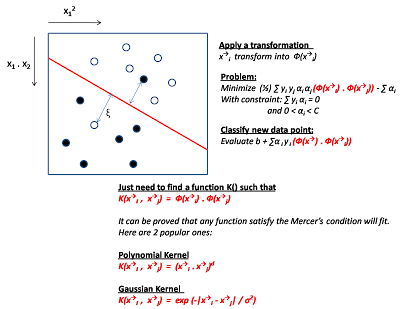## Monday, November 9, 2009

### Support Vector Machine

Support vector machine is a very powerful classification technique. Its theory is based on the linear model but can also handle non-linear model very well. It is also immute to the curse of high dimensionality.

In support vector machine (SVM), inputs are numeric and output are binary. Each data sample can be consider as a m dimension point label as + or - depends on the output.

Optimal separating hyperplane
Assume there are m numeric input attributes, the key approach of SVM is to try finding a (m - 1) dimension hyperplane which can separate the points in the best way. (ie: all the +ve points on one side of the plane and all the -ve points on the other side).

There are many planes that divide the regions. But we need to find the red line which has the maximum margin.Sometimes there may be noise or variation that not all points lies in the same side of the plane. So we modify the equation to allow for some errors in the constraints and we want to minimize the overall errors in the optimization goal.At first glance, it seems like the classification will be O(n) where n is the size of training data. This is not the case because most of the alpha values are zero except the supporting vectors (points touching the margin band) which is a very small value.

Non-Linearity
So far, we have made an assumption that the data is "linearly separable". What if this assumption is not true ? (e.g. y = a1.x1.x1 + a2.x1.x2)

The answer is to create another attribute x3 = x1.x1 and x4 = x1.x2.
In other words, we can always make a non-linear equation becomes linear by introducing extra variables which is a non-linear combination of existing variables. Notice that adding these extra variables effectively is increasing the dimension of the data, but we maintain the linearity of the data point.

As an example, a quadratic equation y = 3x.x + 2x + 5 is a one variable, non-linear equation. But if we introduce z = x.x, then it becomes a 2 variable, linear equation with
y = 3z + 2x + 5

So by adding more attributes to increase the dimensionality of the data points, we can keep the model in linear form. So, we can solve non-linear model by transforming the current data into a higher dimension (adding extra attributes by combining existing attributes in a non-linear way). And then apply the hyperplane separation technique described above to build the model (figure out the alpha value) and use that to classify new data points.

But ! How do we decide what extra attributes should be added and how they should be composed from existing attributes, and how many of them do we need to reconstruct the linearity ?

The Kernal Trick
From examine the above algorithm, an interesting finding is that we only need to know the dot product between two data points but not individual input attribute values. In other words, we don't need to care about how to calculate the extra attributes as long as we know how to calculate the dot product of the new transform space.The process of using SVM is the same as the other machine learning algorithms
1. Pick a tool (such as libSVM)
2. Prepare the input data (convert them to numeric or filter them, normalize their range)
3. Pick a Kernel function and its parameters
4. Run cross-validation against different combination of parameters
5. Pick the best parameter and retrain them.
6. Now we have the learned model, we can use this for classifying new data